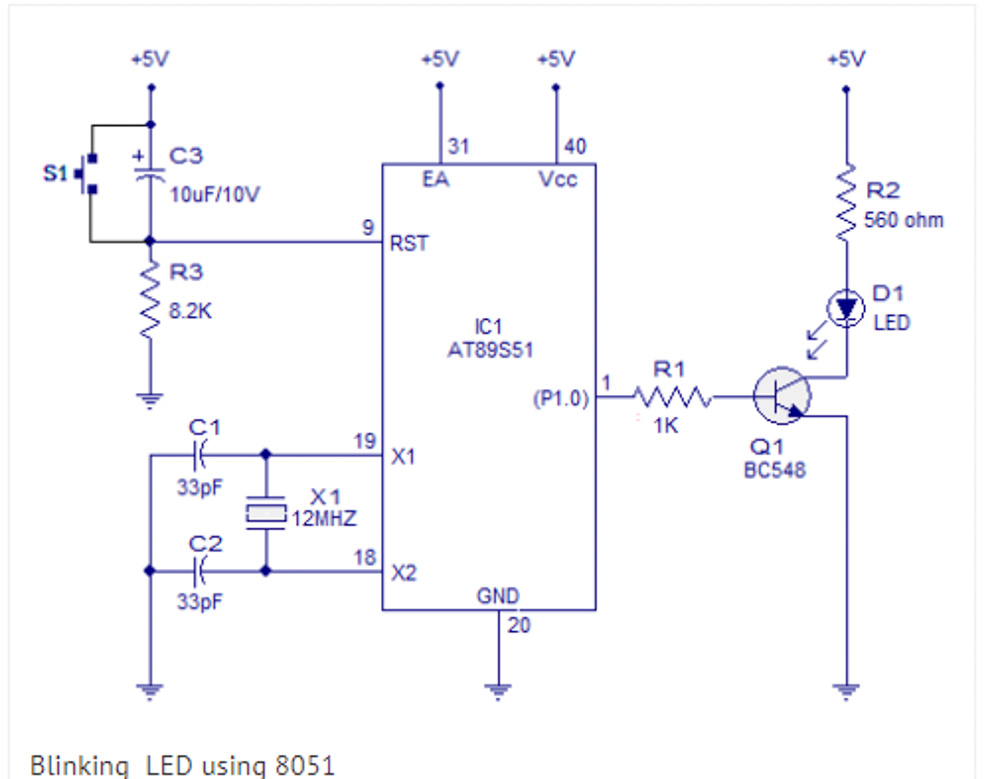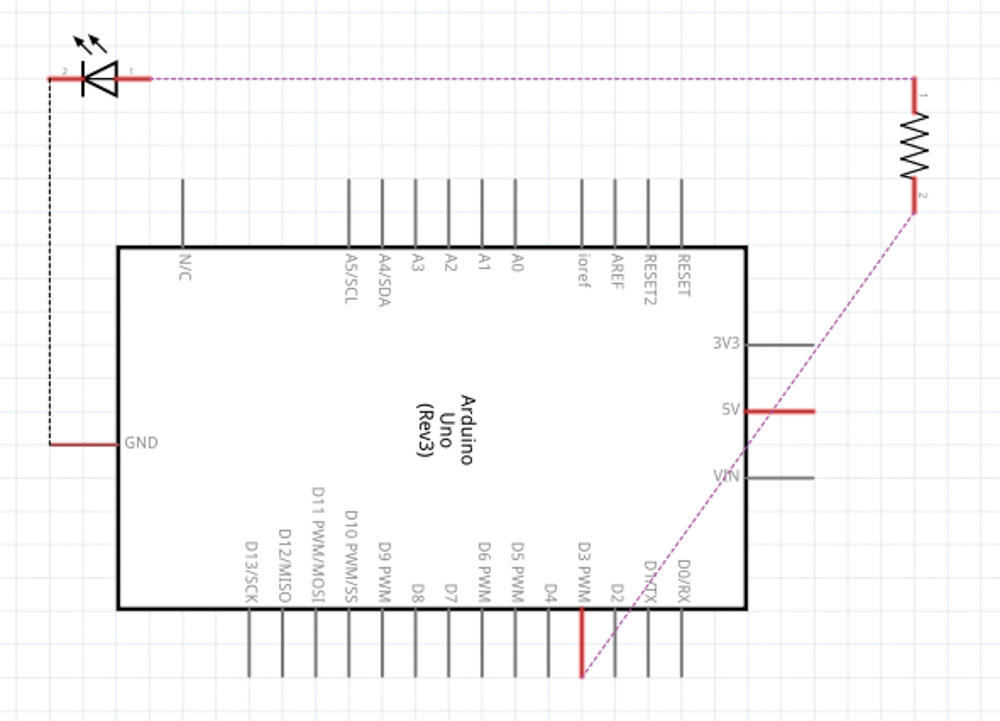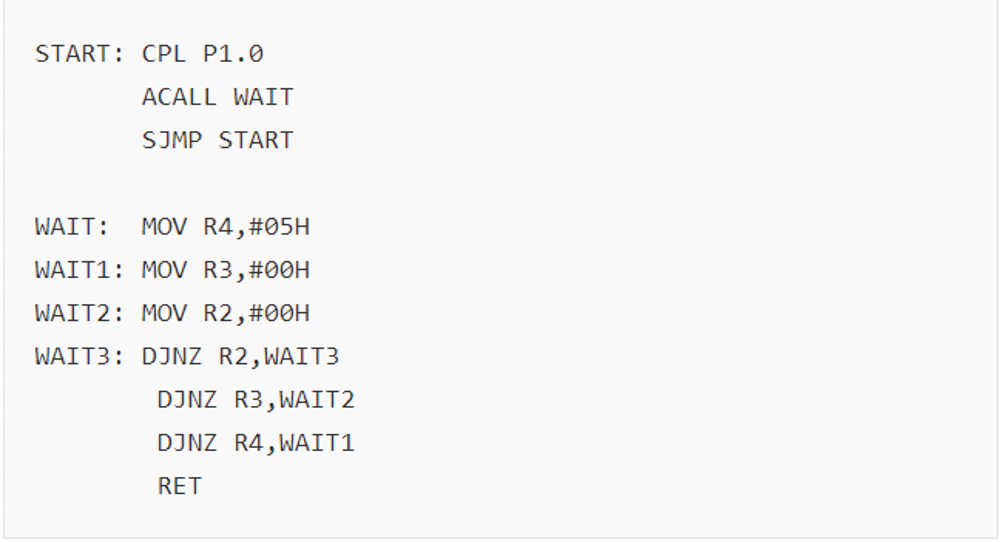8 August 2020

# (Arduino Beginner) Tutorial 0: Introduction to Arduino

## What is an Arduino?

Arduino is an open-source electronics platform based on easy-to-use hardware and software. Basically, it is designed by computer hardware and Software Company OR user community to control or sense objects in the physical world.

## History of Arduino

Arduino was born at Ivrea Interaction Design Institute (IIDI) as an easy tool for fast electronic prototyping, aimed at students without a background in electronics and programming. However, the demand for Arduino design spec started to change after reaching a wider community. For example, changed in 8-bit microcontroller with the 32-bit microcontroller. Today, there are more than 20 different types of official Arduino in the market. (Exclude the mod)## Why using an Arduino?

There are many microcontroller platforms available for physical computing such as Microchip PIC, ARM microcontroller, Intel 8051, Motorola 68HCx series and more. However, these tools take the MESSY details of microcontroller programming. Figures below show the comparison between an Intel 8051 and Arduino on connecting a LED.On the other hand, the Arduino programming block is much easier. Given that![arduino code] (/assets/images/arduino/tutorial/beginner/2020-08-08-tutorial-0/5.jpg)

Arduino offers the following advantages such as:

• Inexpensive hardware
• Support Cross-platform where Arduino IDE runs on Windows, MAC OS and Linux
• Simple and clean environment
• Arduino is open-source hardware where users are free to customize their own board in the mean of improving the performance.

## Arduino basic code block

To truly understand the Arduino code block, here is the example

``````//General Code Block
#include <LiquidCrystal.h>     //This is a library

#define pi 3.142               //Define a Static value
#define Man 5

int i = 2;                     //Define an integer
float j = 2.2;                 //Define a 32 bits precision
double k = 2.2;                //Define a 64 bits precision
char aChar = 'a';              //Define single character
String aString = "Hello";      //Define a string of character
boolean Flag = false;          //Define true or false (1 bit)

void setup()                   //A compulsory function that run once
{
pinMode(i,OUTPUT);           //set pin either Output/input
pinMode(Man,INPUT);
}

void loop()                    //A compulsory function that loop the program
{
digitalWrite(i,HIGH);        //make the output high (5v)
function();
}

void function()                //Not a compulsory function
{
//bla..............
}
``````

## Conclusion

At the end of this course, you will learn:

1. The basic feature of the Arduino board
2. Basic C-programming
3. Basic electronic components
4. Understand the concept of pulse width modulation (PWM)
5. Understand the concept of the analog-to-digital converter (ADC)
6. Arduino application
7. Fun
tags: arduino - beginner - tutorial